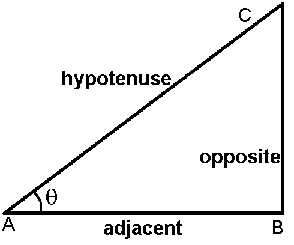# Triangle Angles and Side Calculation Online

Please select the option and enter required values then press calculate button:

 I want to calculate Angle Opposite Side Hypotenuse Side Adjacent Side and Angle Opposite Side Hypotenuse Side Adjacent Side

 Opposite Side : Hypotenuse Side : Adjacent Side : Angle q :[Max 90] Angle b : (90-q) =

Important Formula:

Sin(q) = Opposite / Hypotenuse